Courses

# Series L-R, C-R and C-R Circuit Class 12 Notes | EduRev

## Class 12 : Series L-R, C-R and C-R Circuit Class 12 Notes | EduRev

The document Series L-R, C-R and C-R Circuit Class 12 Notes | EduRev is a part of the Class 12 Course Physics For JEE.
All you need of Class 12 at this link: Class 12

Series L-R Circuit

• Now consider an ac circuit consisting of a resistor of resistance R and an inductor of inductance L in series with an ac source generator.
• Suppose in phasor diagram, current is taken along positive x-direction. The VR is also along positive x-direction and VL along positive y-direction as we know that potential difference across a resistance in ac is in phase with current and it leads in phase by 90º with current across the inductor, and as we know VR = i0R & V0 = i0XL
i = i0 sin wt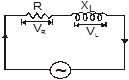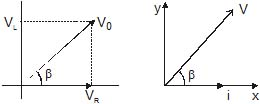VR(t) = i0 Rsin ωt
VL(t) = i0 XL sin (ωt + p/2)
hence we can write
V(t) = i0R sin ωt + i0 XL sin (ωt + p/2)
V0 = i0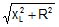whereis known as impedence (z) of the circuit.
now we can write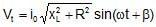where tan β=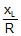hence β =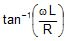Example 1. When 100 volt dc is applied across a coil, a current of 1 amp flows through it; when 100 V ac of 50 Hz is applied to the same coil, only 0.5 amp flows. Calculate the resistance of inductance of the coil.

Sol. In case of a coil, i.e., L - R circuit.

i =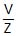with Z =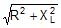=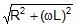So when dc is applied, ω = 0, so z = R

and hence i =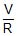i.e., R =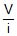=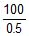= 200 ?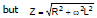i.e., ω2L2 = Z2-R2

i.e., (2πfL)2 = 2002 - 1002 = 3 × 104 (as ω = 2πf)

So, L =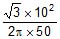=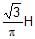= 0.55 H

Example 2. A 12 ohm resistance and an inductance of 0.05/p henry with negligible resistance are connected in series. Across the end of this circuit is connected a 130 volt alternating voltage of frequency 50 cycles/second. Calculate the alternating current in the circuit and potential difference across the resistance and that across the inductance.

Sol. The impedance of the circuit is given by

Z =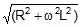=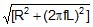=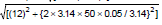=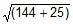= 13 ohm

Current in the circuit i = E/Z =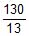= 10 amp

Potential difference across resistance

VR = iR = 10 × 12 = 120 volt

Inductive reactance of coil XL = ωL = 2πfL

Therefore, XL = 2π × 50 ×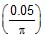= 5 ohm

Potential difference across inductance

VL = i × XL = 10 × 5 = 50 volt

Series C-R Circuit

Now consider an ac circuit consisting of a resistor of resistance R and an capacitor of capacitance C in series with an ac source generator.

Suppose in phasor diagram current is taken along positive x-direction. Then VR is also along positive x-direction but VC is along negative y-direction as potential difference across a capacitor in ac lags in phase by 90º with the current in the circuit. So we can write,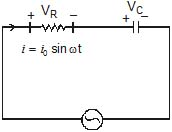VR = I0 R sin ωt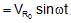Potential difference across capacitor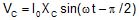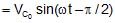Potential at any instant t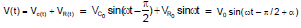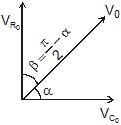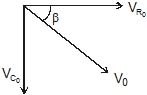V(t) = V0 sin (ωt + b)

tan α =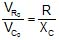Example 3. An A.C. source of angular frequency w is fed across a resistor R and a capacitor C in series. The current registered is i. If now the frequency of the source is changed to ω/3 (but maintaining the same voltage), the current in the circuit is found to be halved. Calculate the ratio of reactance to resistance at the original frequency.

Sol. At angular frequency w, the current in R-C circuit is given by

irms =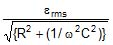...(i)

When frequency is changed to ω/3, the current is halved. Thus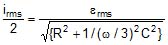=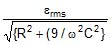...(ii)

From equations (i) and (ii), we have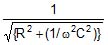=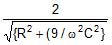Solving this equation, we getHence, the ratio of reactance to resistance is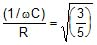Example 4. A 50 W, 100 V lamp is to be connected to an ac mains of 200 V, 50 Hz. What capacitance is essential to be put in series with the lamp?

Sol. As resistance of the lamp R =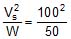= 200 ? and the maximum current i ==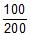=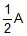; so when the lamp is put in series with a capacitance and run at 200 V ac, from V =iZ we have,

Z ==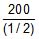= 400?

Now as in case of C-R circuit, Z =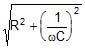,

i.e., R2 +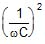= 160000

or,= 16 × 104 - (200)2 = 12 × 104

So,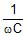=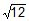× 102

or C =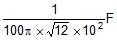i.e., C =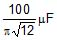= 9.2 μF

L.C. Circuit

As shown in figure a capacitor and inductance are connected in series method and alternating voltage is applied across the circuit.

Let Xc is capacitance reactance,

XL is Inductance reactance,

i = i0 sin wt current flowing through the circuit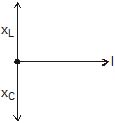VC(t) = i0 XC sin (ωt - π/2)

VL(t) = i0 XL sin (ωt + π/2)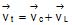= i0 XC sin wt cos π/2 - i0 XC cos wt sin π/2 + i0 XL sin wt cos π/2 + i0 XL cos wt sin π/2

= i0 cos w t(XL - XC)

V(t) = V0sin (wt + p/2)

V0 = i0Z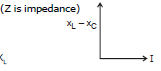Z = (X- XC)

cos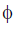= 0

VCO = i0 XC ;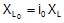Series L-C-R Circuit

Now consider an ac circuit consisting of a resistor of resistance R, a capacitor of capacitance C and an inductor of inductance L are in series with an ac source generator.

Suppose in a phasor diagram current is taken along positive x-direction. Then VR is along positive x-direction, VL along positive y-direction and VC along negative y-direction, as potential difference across an inductor leads the current by 90° in phase while that across a capacitor, lags by 90°.

V =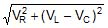L - R - C circuit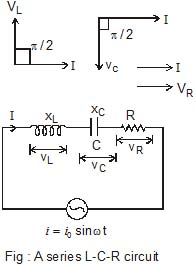Impedance phasor of above circuit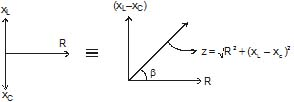& Impedance triangle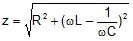here B is phase angle By triangle tan β=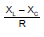Power factor cos=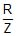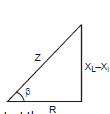Let I be the current in the series circuit of any instant then

(1) Voltage V(t) = V0 sin (ωt + β) = i0 z sin (ωt + β)

here v0 = i0z & vrms = irmsz

(2)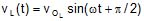here voltage VL across the inductance is ahead of current I in phase by π/2 rad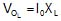(3) VC(t) = VO C sin (ωt - π/2)

here voltage VC across the capacitance lags behind the current I in phase by π/2 rad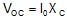(4) VR(t) = i0 R sin ωt

here voltage VR across the resistor R has same phase as I

VO R = IR

Special Case :

(1) When XL > XC or VL > VC then emf is ahead of current by phase β which is given by

tan β =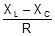or cos f =The series LCR circuit is said to be inductive

(2) When XL < XC or VL < VC then current is ahead of emf by phase angle β which is given by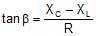or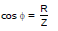The series LCR circuit is said to be capacitive

(3) When XL = XC or VL = VC, b = 0, the emf and current will be in the same phase. The series LCR circuit is said to be purely resistive. It may also be noted that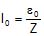or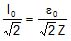or IRms =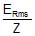Susceptance : The reciprocal of the reactane of an a.c. circuit is called its susceptance.

Admittance : The reciprocal of the impedance of an a.c. circuit is called its admittance.

Ex.10 Figure shows a series LCR circuit connected to a variable voltage source V = 10 sin (ωt + p/4) ;

xL = 10 ?, XC = 6 ?, R = 3 ?

Calculate Z, i0, irms, vrms, VL O, VC O, VR O, b,

VL Rms, VC Rms, VRms, i(t), VL(t), Vc(t), and VR(t)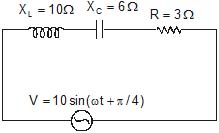XL > XC

Sol. V = 10 sin (wt + p/4) so V0 = 10 volt

Vrms =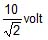Therefore, Z =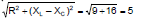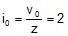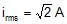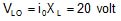;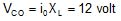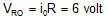;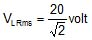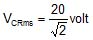;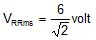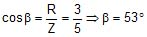i(t) = 20 sin (ωt + π/4 - 53°)

VL(t) = 20 sin (ωt + π/4 - 53° + π/2)

= 20 sin (ωt +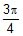- 53° -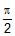) = 12 sin (ωt -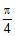- 53°)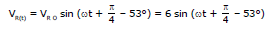Ex.11 A resistor of resistance R, an inductor of inductance L and a capacitor of capacitance C all are connected in series with an a.c. supply. The resistance of R is 16 ohm and for a given frequency the inductive reactance of L is 24 ohm and capacitive reactance of C is 12 ohm. If the current in the circuit is 5 amp., find

(a) the potential difference across R, L and C (b) the impedance of the circuit

(c) the voltage of a.c. supply (d) phase angle

Sol. (a) Potential difference across resistance

VR = iR = 5 × 16 = 80 volt

Potential difference across inductance

VL = i × (wL) = 5 × 24 = 120 volt

Offer running on EduRev: Apply code STAYHOME200 to get INR 200 off on our premium plan EduRev Infinity!

## Physics For JEE

187 videos|516 docs|263 tests

,

,

,

,

,

,

,

,

,

,

,

,

,

,

,

,

,

,

,

,

,

,

,

,

;